# Curved MirrorsPage 1

#### WATCH ALL SLIDES

Slide 1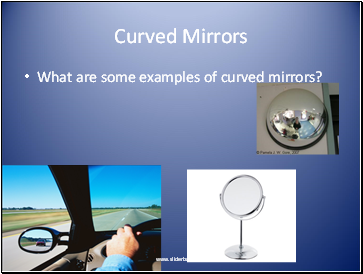## Curved Mirrors

What are some examples of curved mirrors?

Slide 2## Concave Spherical Mirrors

A spherical mirror has the shape of part of a sphere’s surface.

Concave Spherical Mirror (Converging Mirror): A spherical mirror with light reflecting from its silvered, concave surface

Concave mirrors are used whenever a magnified image of an object is needed

Slide 3Terms you need to know

Slide 4Concave Spherical Mirrors (p.531)

A spherical mirror is represented as a shell of a sphere

Slide 5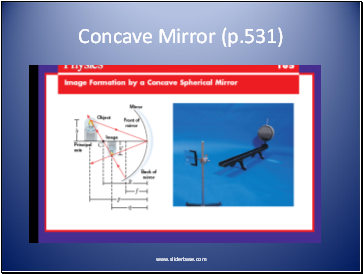Concave Mirror (p.531)

Slide 6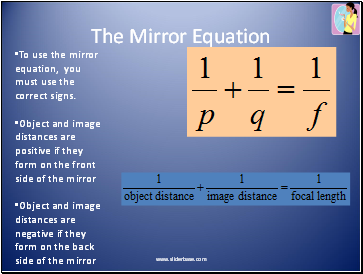## The Mirror Equation

To use the mirror equation, you must use the correct signs.

Object and image distances are positive if they form on the front side of the mirror

Object and image distances are negative if they form on the back side of the mirror

Slide 7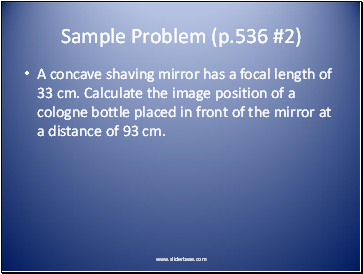Sample Problem (p.536 #2)

A concave shaving mirror has a focal length of 33 cm. Calculate the image position of a cologne bottle placed in front of the mirror at a distance of 93 cm.

Slide 8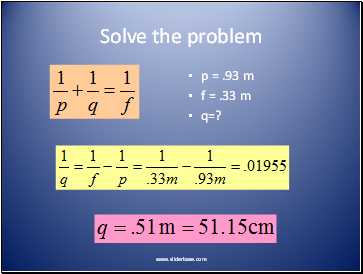Solve the problem

p = .93 m

f = .33 m

q=?

Slide 9Magnification Equation

Slide 10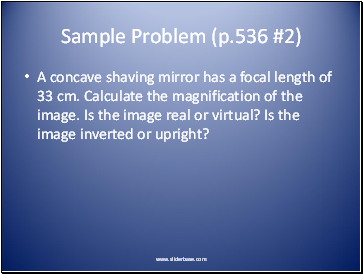## Sample Problem (p.536 #2)

A concave shaving mirror has a focal length of 33 cm. Calculate the magnification of the image. Is the image real or virtual? Is the image inverted or upright?

Slide 11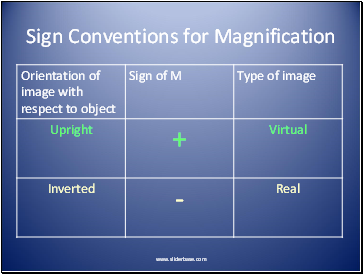Sign Conventions for Magnification

Slide 12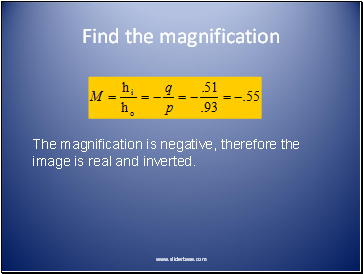Find the magnification

The magnification is negative, therefore the image is real and inverted.

Slide 13## Ray Diagrams

A ray diagram is a drawing that uses geometry to locate an image formed by a mirror.

There are different rules for drawing ray diagrams depending on the type of mirror you have.

Slide 14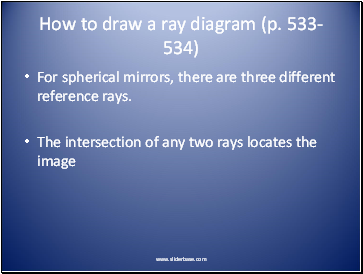Go to page:
1  2  3